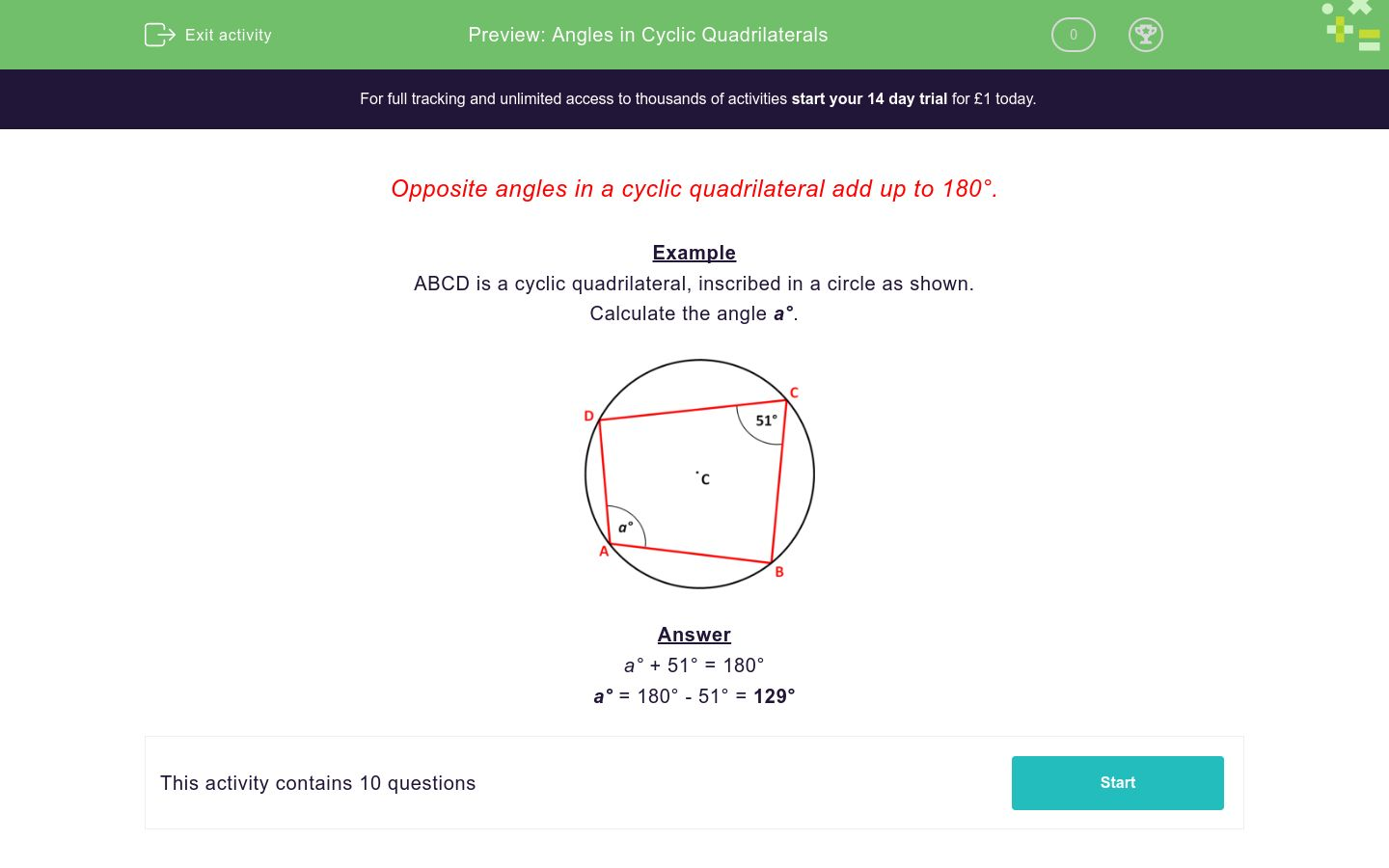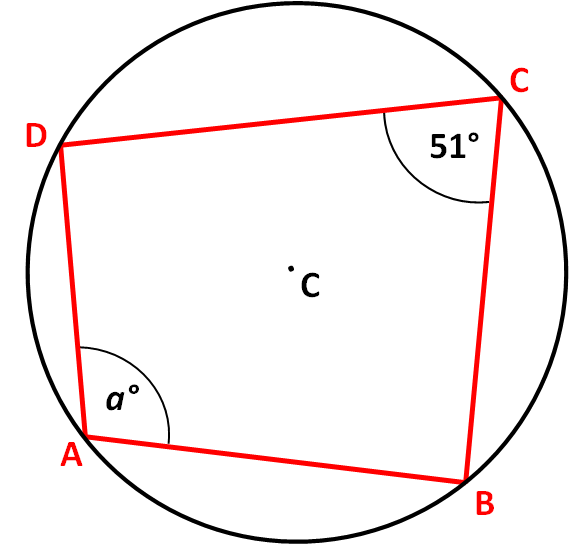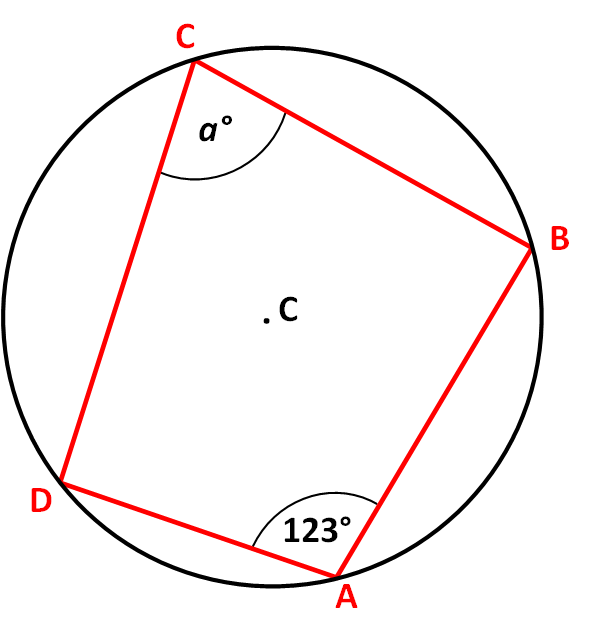# Angles in Cyclic Quadrilaterals

In this worksheet, students must find missing angles in inscribed quadrilaterals.Key stage:  KS 4

Curriculum topic:  Geometry and Measures

Curriculum subtopic:  Identify and Apply Circle Definitions and Properties

Difficulty level:### QUESTION 1 of 10

Opposite angles in a cyclic quadrilateral add up to 180°.

Example

ABCD is a cyclic quadrilateral, inscribed in a circle as shown.

Calculate the angle .+ 51° = 180°

= 180° - 51° = 129°

ABCD is a cyclic quadrilateral, inscribed in a circle as shown.

Calculate the angle .

Just write the number.Not drawn accurately.

ABCD is a cyclic quadrilateral, inscribed in a circle as shown.

Calculate the angle .

Just write the number.Not drawn accurately.

ABCD is a cyclic quadrilateral, inscribed in a circle as shown.

Calculate the angle .

Just write the number.Not drawn accurately.

ABCD is a cyclic quadrilateral, inscribed in a circle as shown.

Calculate the angle .

Just write the number.Not drawn accurately.

ABCD is a cyclic quadrilateral, inscribed in a circle as shown.

Calculate the angle .

Just write the number.Not drawn accurately.

ABCD is a cyclic quadrilateral, inscribed in a circle as shown.

Calculate the angle .

Just write the number.Not drawn accurately.

ABCD is a cyclic quadrilateral, inscribed in a circle as shown.

Calculate the angle .

Just write the number.Not drawn accurately.

ABCD is a cyclic quadrilateral, inscribed in a circle as shown.

Calculate the angle .

Just write the number.Not drawn accurately.

ABCD is a cyclic quadrilateral, inscribed in a circle as shown.

Calculate the angle .

Just write the number.Not drawn accurately.

ABCD is a cyclic quadrilateral, inscribed in a circle as shown.

Calculate the angle .

Just write the number.Not drawn accurately.

• Question 1

ABCD is a cyclic quadrilateral, inscribed in a circle as shown.

Calculate the angle .

Just write the number.Not drawn accurately.

117
EDDIE SAYS
a° = 180° - 63°
• Question 2

ABCD is a cyclic quadrilateral, inscribed in a circle as shown.

Calculate the angle .

Just write the number.Not drawn accurately.

69
EDDIE SAYS
a° = 180° - 111°
• Question 3

ABCD is a cyclic quadrilateral, inscribed in a circle as shown.

Calculate the angle .

Just write the number.Not drawn accurately.

81
EDDIE SAYS
a° = 180° - 99°
• Question 4

ABCD is a cyclic quadrilateral, inscribed in a circle as shown.

Calculate the angle .

Just write the number.Not drawn accurately.

85
EDDIE SAYS
a° = 180° - 95°
• Question 5

ABCD is a cyclic quadrilateral, inscribed in a circle as shown.

Calculate the angle .

Just write the number.Not drawn accurately.

65
EDDIE SAYS
a° = 180° - 115°
• Question 6

ABCD is a cyclic quadrilateral, inscribed in a circle as shown.

Calculate the angle .

Just write the number.Not drawn accurately.

57
EDDIE SAYS
a° = 180° - 123°
• Question 7

ABCD is a cyclic quadrilateral, inscribed in a circle as shown.

Calculate the angle .

Just write the number.Not drawn accurately.

93
EDDIE SAYS
a° = 180° - 87°
• Question 8

ABCD is a cyclic quadrilateral, inscribed in a circle as shown.

Calculate the angle .

Just write the number.Not drawn accurately.

109
EDDIE SAYS
a° = 180° - 71°
• Question 9

ABCD is a cyclic quadrilateral, inscribed in a circle as shown.

Calculate the angle .

Just write the number.Not drawn accurately.

48
EDDIE SAYS
a° = 180° - 132°
• Question 10

ABCD is a cyclic quadrilateral, inscribed in a circle as shown.

Calculate the angle .

Just write the number.Not drawn accurately.